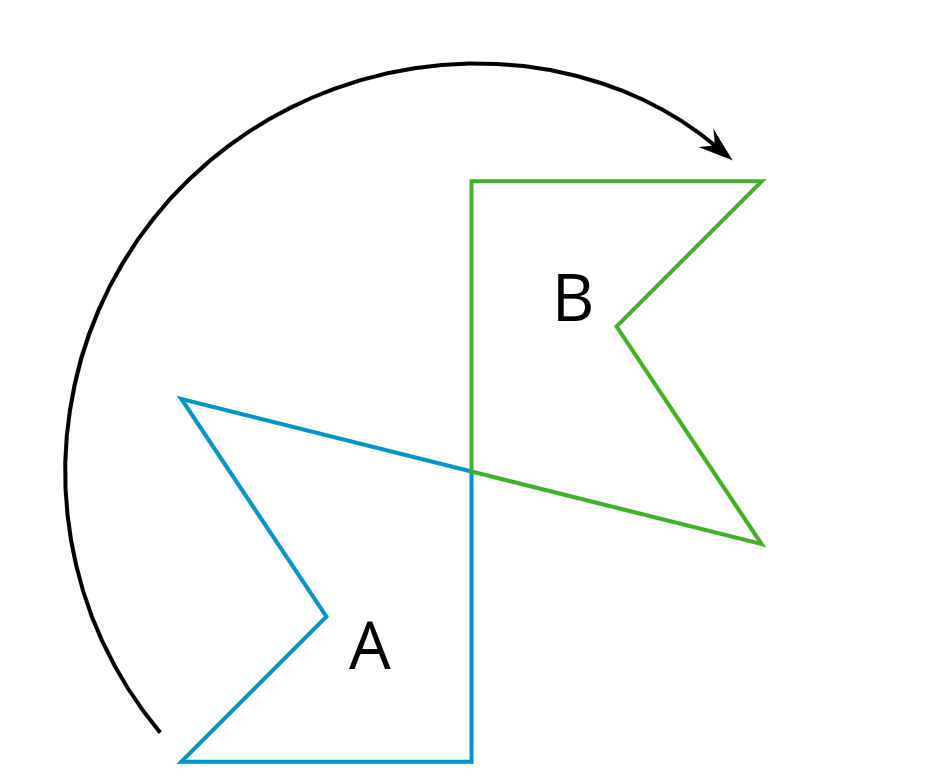# Lesson 4

Making the Moves

### Lesson Narrative

In the previous lesson, students were introduced to the terms “translation,” “rotation,” and “reflection.” In this lesson, students understand that:

• A translation is determined by two points that specify the distance and direction of the translation.
• A rotation is determined by a point, angle of rotation, and a direction of rotation.
• A reflection is determined by a line.

These moves are called transformations for the first time and students draw images of figures under these transformations. They also study where shapes go under sequences of these transformations and identify the steps in a sequence of transformations that takes one figure to another. Note the subtle shift in language. In the previous lesson, one shape “moves” to the other shape—it is as if the original shape has agency and does the moving. In this lesson, the transformation “takes” one shape to the other shape—this language choice centers the transformation itself as an object of study.

Students using the print version may make use of tracing paper to experiment moving shapes. Students using the digital version have access to geogebra applets with which to perform transformations. Whenever students choose to make use of an appropriate tool, they are engaging in MP5. Students are also likely starting to begin thinking strategically about which transformations will take one figure to another, identifying properties of the shapes that indicate whether a translation, rotation, reflection or sequence of these will achieve this goal (MP7).

### Learning Goals

Teacher Facing

• Comprehend that a “transformation” is a translation, rotation, reflection, or a combination of these.
• Draw a transformation of a figure using information given orally.
• Explain (orally) the “sequence of transformations” that “takes” one figure to its image.
• Identify (orally and in writing) the features that determine a translation, rotation, or reflection.

### Student Facing

Let’s draw and describe translations, rotations, and reflections.

### Required Preparation

Print and cut up cards from the Make that Move blackline master. Prepare 1 set of cards for every 4 students.

### Student Facing

• I can use the terms translation, rotation, and reflection to precisely describe transformations.

### Glossary Entries

• clockwise

Clockwise means to turn in the same direction as the hands of a clock. The top turns to the right. This diagram shows Figure A turned clockwise to make Figure B.• counterclockwise

Counterclockwise means to turn opposite of the way the hands of a clock turn. The top turns to the left.

This diagram shows Figure A turned counterclockwise to make Figure B.

• image

An image is the result of translations, rotations, and reflections on an object. Every part of the original object moves in the same way to match up with a part of the image.

In this diagram, triangle $$ABC$$ has been translated up and to the right to make triangle $$DEF$$. Triangle $$DEF$$ is the image of the original triangle $$ABC$$.

• reflection

A reflection across a line moves every point on a figure to a point directly on the opposite side of the line. The new point is the same distance from the line as it was in the original figure.

This diagram shows a reflection of A over line $$\ell$$ that makes the mirror image B.

• rotation

A rotation moves every point on a figure around a center by a given angle in a specific direction.

This diagram shows Triangle A rotated around center $$O$$ by 55 degrees clockwise to get Triangle B.

• sequence of transformations

A sequence of transformations is a set of translations, rotations, reflections, and dilations on a figure. The transformations are performed in a given order.

This diagram shows a sequence of transformations to move Figure A to Figure C.

First, A is translated to the right to make B. Next, B is reflected across line $$\ell$$ to make C.

• transformation

A transformation is a translation, rotation, reflection, or dilation, or a combination of these.

• translation

A translation moves every point in a figure a given distance in a given direction.

This diagram shows a translation of Figure A to Figure B using the direction and distance given by the arrow.

The vertices in this polygon are labeled $$A$$, $$B$$, $$C$$, $$D$$, and $$E$$.